#Function Repository Resource:

# Fibbinary

Get the fibbinary sequence

Contributed by: Wolfram staff, based on work by Robert G. Wilson, Eric W. Weisstein
 ResourceFunction["Fibbinary"][n] gives the nth fibbinary number. ResourceFunction["Fibbinary"][{n}] gives the fibbinary numbers until n.

## Details and Options

Fibbinary numbers are the positive integers whose binary representation contains no consecutive ones.

## Examples

### Basic Examples

First 100 fibbinaries:

 In:=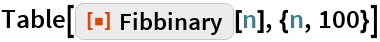Out=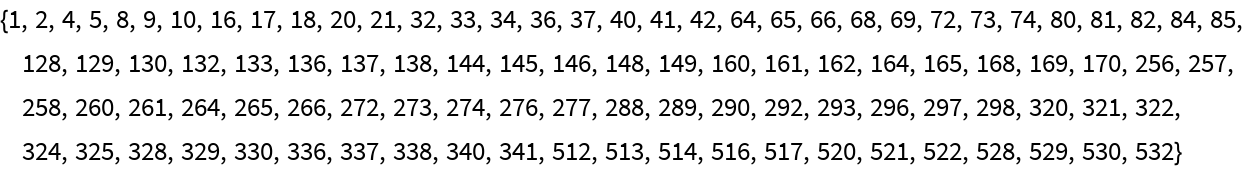Fibbinaries until 50 in base 2:

 In:=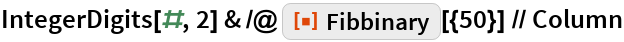Out=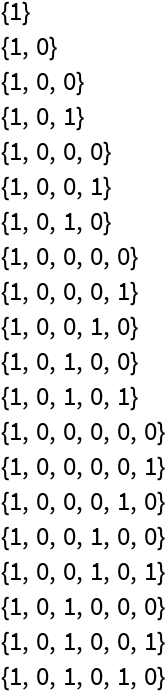Plot of fibbinaries until 150 in base 2:

 In:=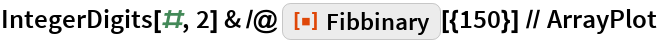Out=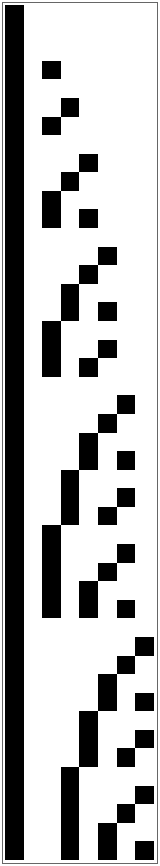### Properties and Relations

Fibbinaries are related to the Zeckendorf representation:

 In:=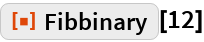Out=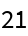In:=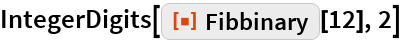Out=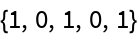In:=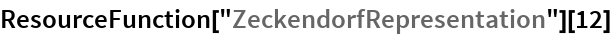Out=Adding the following integers gives 12:

 In:=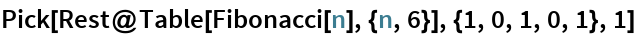Out=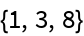## Requirements

Wolfram Language 11.3 (March 2018) or above